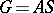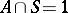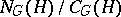# Normal p-complement

(diff) ← Older revision | Latest revision (diff) | Newer revision → (diff)
Jump to: navigation, search

of a finite groupA normal subgroupsuch thatand, whereis a Sylow-subgroup of(see Sylow subgroup). A grouphas a normal-complement if some Sylow-subgroupoflies in the centre of its normalizer (cf. Normalizer of a subset) (Burnside's theorem). A necessary and sufficient condition for the existence of a normal-complement in a groupis given by Frobenius' theorem: A grouphas a normal-complement if and only either for any non-trivial-subgroupofthe quotient groupis a-group (whereis the normalizer andthe centralizer ofin) or if for every non-trivial-subgroupofthe subgrouphas a normal-complement.